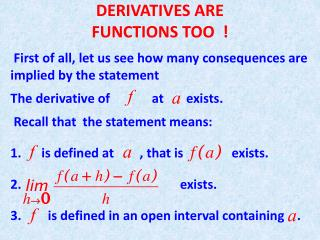Download PresentationDERIVATIVES ARE FUNCTIONS TOO !

# DERIVATIVES ARE FUNCTIONS TOO ! - PowerPoint PPT PresentationDownload Presentation## DERIVATIVES ARE FUNCTIONS TOO !

- - - - - - - - - - - - - - - - - - - - - - - - - - - E N D - - - - - - - - - - - - - - - - - - - - - - - - - - -
##### Presentation Transcript

1. DERIVATIVES AREFUNCTIONS TOO ! First of all, let us see how many consequences are implied by the statement The derivative of at exists. Recall that the statement means: • is defined at , that is exists. • exists. • is defined in an open interval containing .

2. If we change the symbol to something else, say (it’s a free country !), we still get the notion of the derivative of at , namely . Now,if we change the symbol to something else, say (it’s a free country !), we get the notion of the derivative of at as another function, variously denoted by

3. So, given a function we have manufactured a new one, . Note that if denotes the domain of , the domain of may be smaller (missing those points where does NOT have a derivative, we’ll find a few soon.) There are deep relationships between and . Here is one that is simply defining a word: If exists we say that

4. is differentiable at . (We could have said that is “well-behaved at “ , or “cool at “ or something else, but the convention is to say it is differentiable, must have to do with the fact that the limit of the difference quotient exists!) Here is a first important (if trivial) consequence of differentiability. Theorem. If is differentiable at then is continuous at . Proof. (We use purely mathematical language)

6. You have seen the verification of the following trivial statement (in everyday language) If a fraction has a finite limit, and the bottom goes to 0, the top has to go to 0 also. (Duh!) The theorem we just proved helps us decide where in its domain a function might not be differentiable, namely at any point where continuity fails. Any other places where differentiability might not be? Let’s see …. The definition says

7. differentiable at means that exists. So both and exist and are equal. Therefore differentiability fails at any where (naturally we assume continuity at .)

8. A. Either one or both of the one-sided limits fail to exist or B. Both exist but are not equal Remark. We will give examples where situation A obtains and also where situation B obtains. In fact it’s easy to verify that for the function the left-hand limit is , the right-hand DNE !

9. We can see trivially that and it’s easy to show that Quick proof: The graph of the function is shown in the next slide

10. The graph The next graph has both one-sided limits failing to exist.

11. The graph of Both one-sided limits are (– on left) Finally, the function

12. has graph

13. and this time while i.e both one-sided limits exist but are not equal. Let’s get back to nice functions that are differentiable. What we have in this case is that a function gives rise to another function, namely .

14. We can represent pictorially this situation with the following diagram We say that is the derivative of .

15. Of course we can play the game again, to get the Derivative of the derivative, usually called the second derivative, and denoted variously by As they say in Casablanca, play it again Sam, we’ll get the third derivative, denoted by

16. Why stop when we’re having fun. Let’s go for the fourth derivative, the fifth, and so on … Right now our trouble is that we officially don’t know how to compute derivatives, other than by the laborious method of applying the definition! Let’s compute the first derivative of

17. Here we go. Much to the chagrin of your High School Math teacher, what changes here is , not ! Now Do some 7th grade algebra to get

18. (after you have cleared the smoke!) and therefore Clearly we need a few bricks and mortar to build our differentiation edifice! Stay tuned folks!